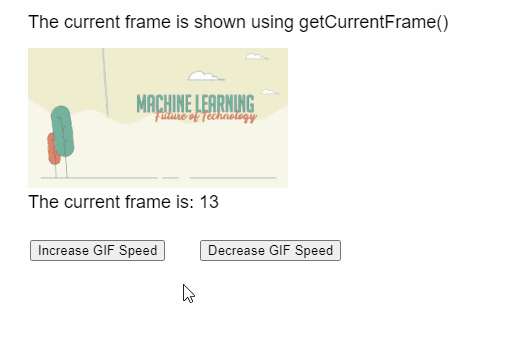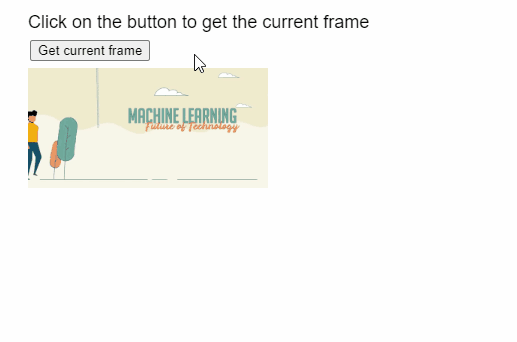GeeksforGeeks App
Open AppBrowser
Continue

# p5.js Image getCurrentFrame() Method

The getCurrentFrame() method of p5.Image in p5.js library is used to return the index of the currently shown frame of the GIF animation.

Syntax:

`  getCurrentFrame()`

Parameters: This function accept does not accept any parameter.

Return Value: This method returns a number that represents the current frame of the animation that is currently visible.

The following libraries are included in the “head” section of the HTML page while implementing the following examples.

<script src=”p5.Image.js”></script>
<script src=”p5.min.js”></script>

Example 1: The example below illustrates the getCurrentFrame() method in p5.js library.

## Javascript

 `function` `setup() {``  ``example_gif =``    ``loadImage(``"sample-gif.gif"``);` `  ``createCanvas(500, 300);``  ``textSize(18);` `  ``incSpeedBtn =``    ``createButton(``"Increase GIF Speed"``);``  ``incSpeedBtn.position(30, 240);``  ``incSpeedBtn.mousePressed(() => {` `      ``// Decrease the delay between frames``      ``example_gif.delay(25);``  ``})` `  ``decSpeedBtn =``    ``createButton(``"Decrease GIF Speed"``);``  ``decSpeedBtn.position(200, 240);``  ``decSpeedBtn.mousePressed(() =>`` ``{``      ``// Increase the delay between frames``      ``example_gif.delay(150);``  ``})``}` `function` `draw()``{``  ``clear();` `  ``text(``"The current frame is shown "` `+``       ``"using getCurrentFrame()"``, 20, 20);``       ` `  ``// Draw the GIF on screen``  ``image(example_gif, 20, 40, 260, 140);` `  ``// Get the current frame``  ``let currFrame =``      ``example_gif.getCurrentFrame();` `  ``text(``"The current frame is: "` `+``       ``currFrame, 20, 200);``}`

Output:Example 2:

## Javascript

 `function` `preload() {``  ``example_gif =``    ``loadImage(``"sample-gif.gif"``);``}` `function` `setup() {``  ``createCanvas(500, 300);``  ``textSize(18);` `  ``text(``"Click on the button to get "` `+``       ``"the current frame"``, 20, 20);` `  ``frameBtn =``    ``createButton(``"Get current frame"``);``  ``frameBtn.position(30, 40);``  ``frameBtn.mousePressed(getFrame);``}` `function` `draw() {``  ``// Draw the GIF on screen``  ``image(example_gif, 20, 60, 240, 120);``}` `function` `getFrame()``{``  ``clear();` `  ``// Get the current frame``  ``let currFrame =``      ``example_gif.getCurrentFrame();` `  ``text(``"The current frame is: "` `+``       ``currFrame, 20, 200);` `  ``text(``"Click on the button to get "` `+``       ``"the current frame"``, 20, 20);``}`

Output:Online editor: https://editor.p5js.org/
Environment Setup: https://www.geeksforgeeks.org/p5-js-soundfile-object-installation-and-methods/
Reference: https://p5js.org/reference/#/p5.Image/getCurrentFrame

My Personal Notes arrow_drop_up
Related Tutorials